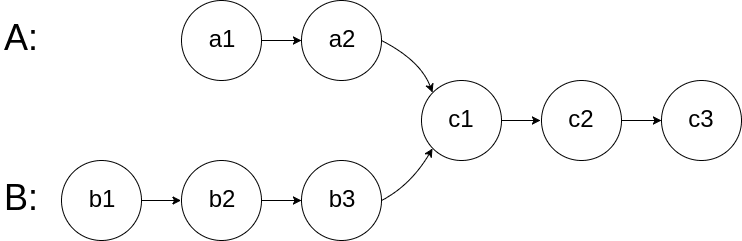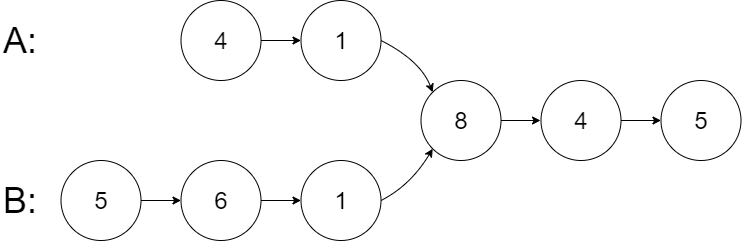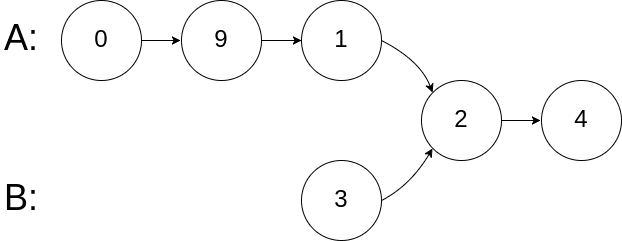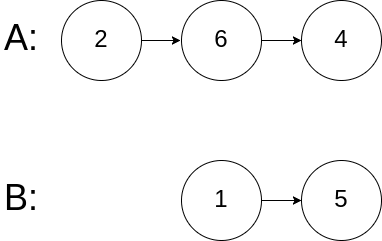# Question

Formatted question description: https://leetcode.ca/all/160.html

Given the heads of two singly linked-lists headA and headB, return the node at which the two lists intersect. If the two linked lists have no intersection at all, return null.

For example, the following two linked lists begin to intersect at node c1:The test cases are generated such that there are no cycles anywhere in the entire linked structure.

Note that the linked lists must retain their original structure after the function returns.

Custom Judge:

The inputs to the judge are given as follows (your program is not given these inputs):

• intersectVal - The value of the node where the intersection occurs. This is 0 if there is no intersected node.
• listA - The first linked list.
• listB - The second linked list.
• skipA - The number of nodes to skip ahead in listA (starting from the head) to get to the intersected node.
• skipB - The number of nodes to skip ahead in listB (starting from the head) to get to the intersected node.

The judge will then create the linked structure based on these inputs and pass the two heads, headA and headB to your program. If you correctly return the intersected node, then your solution will be accepted.

Example 1:Input: intersectVal = 8, listA = [4,1,8,4,5], listB = [5,6,1,8,4,5], skipA = 2, skipB = 3
Output: Intersected at '8'
Explanation: The intersected node's value is 8 (note that this must not be 0 if the two lists intersect).
From the head of A, it reads as [4,1,8,4,5]. From the head of B, it reads as [5,6,1,8,4,5]. There are 2 nodes before the intersected node in A; There are 3 nodes before the intersected node in B.
- Note that the intersected node's value is not 1 because the nodes with value 1 in A and B (2nd node in A and 3rd node in B) are different node references. In other words, they point to two different locations in memory, while the nodes with value 8 in A and B (3rd node in A and 4th node in B) point to the same location in memory.


Example 2:Input: intersectVal = 2, listA = [1,9,1,2,4], listB = [3,2,4], skipA = 3, skipB = 1
Output: Intersected at '2'
Explanation: The intersected node's value is 2 (note that this must not be 0 if the two lists intersect).
From the head of A, it reads as [1,9,1,2,4]. From the head of B, it reads as [3,2,4]. There are 3 nodes before the intersected node in A; There are 1 node before the intersected node in B.


Example 3:Input: intersectVal = 0, listA = [2,6,4], listB = [1,5], skipA = 3, skipB = 2
Output: No intersection
Explanation: From the head of A, it reads as [2,6,4]. From the head of B, it reads as [1,5]. Since the two lists do not intersect, intersectVal must be 0, while skipA and skipB can be arbitrary values.
Explanation: The two lists do not intersect, so return null.


Constraints:

• The number of nodes of listA is in the m.
• The number of nodes of listB is in the n.
• 1 <= m, n <= 3 * 104
• 1 <= Node.val <= 105
• 0 <= skipA < m
• 0 <= skipB < n
• intersectVal is 0 if listA and listB do not intersect.
• intersectVal == listA[skipA] == listB[skipB] if listA and listB intersect.

Follow up: Could you write a solution that runs in O(m + n) time and use only O(1) memory?

# Algorithm

If the two chains are the same length, then the corresponding ones can be found one by one, so you only need to shorten the long list.

The specific algorithm is: traverse the two linked lists respectively to obtain the corresponding lengths. Then find the difference in length, move the longer linked list backward by the number of differences, and then compare them one by one.

# Code

• 
/**
* public class ListNode {
*     int val;
*     ListNode next;
*     ListNode(int x) {
*         val = x;
*         next = null;
*     }
* }
*/

/**
* public class ListNode {
*     int val;
*     ListNode next;
*     ListNode(int x) {
*         val = x;
*         next = null;
*     }
* }
*/

public class Solution {

while (lenA > lenB) {
lenA--;
}
while (lenA < lenB) {
lenB--;
}

// find the intersection until end
}
}

private int getLength(ListNode node) {
int length = 0;
while (node != null) {
node = node.next;
length++;
}
return length;
}
}
}

############

/**
* public class ListNode {
*     int val;
*     ListNode next;
*     ListNode(int x) {
*         val = x;
*         next = null;
*     }
* }
*/
public class Solution {
while (a != b) {
a = a == null ? headB : a.next;
b = b == null ? headA : b.next;
}
return a;
}
}

• // OJ: https://leetcode.com/problems/intersection-of-two-linked-lists/
// Time: O(A + B)
// Space: O(1)
class Solution {
int getLength(ListNode *h) {
int len = 0;
for (; h; h = h->next) ++len;
return len;
}
public:
ListNode *getIntersectionNode(ListNode *a, ListNode *b) {
int la = getLength(a), lb = getLength(b);
if (la < lb) swap(a, b), swap(la, lb);
for (int i = la - lb; i > 0; --i) a = a->next;
while (a && b && a != b) a = a->next, b = b->next;
return a;
}
};

• # Definition for singly-linked list.
# class ListNode:
#     def __init__(self, x):
#         self.val = x
#         self.next = None

class Solution:
while a != b:
a = a.next if a else headB
b = b.next if b else headA
return a

############

class Solution:
return None
while pa is not pb:
pa = headB if pa is None else pa.next
pb = headA if pb is None else pb.next
return pa


• /**
* type ListNode struct {
*     Val int
*     Next *ListNode
* }
*/
for a != b {
if a == nil {
} else {
a = a.Next
}
if b == nil {
} else {
b = b.Next
}
}
return a
}

• /**
* class ListNode {
*     val: number
*     next: ListNode | null
*     constructor(val?: number, next?: ListNode | null) {
*         this.val = (val===undefined ? 0 : val)
*         this.next = (next===undefined ? null : next)
*     }
* }
*/

function getIntersectionNode(
): ListNode | null {
while (a != b) {
a = a ? a.next : headB;
b = b ? b.next : headA;
}
return a;
}


• /**
* function ListNode(val) {
*     this.val = val;
*     this.next = null;
* }
*/

/**
* @return {ListNode}
*/
while (a != b) {
a = a ? a.next : headB;
b = b ? b.next : headA;
}
return a;
};


• /**
* public class ListNode {
*     public var val: Int
*     public var next: ListNode?
*     public init(_ val: Int) {
*         self.val = val
*         self.next = nil
*     }
* }
*/

class Solution {